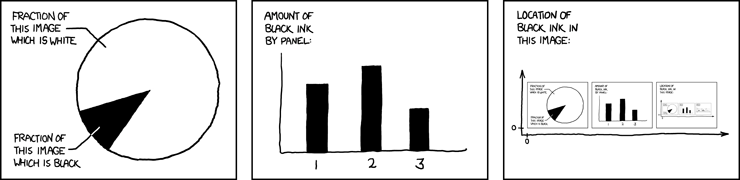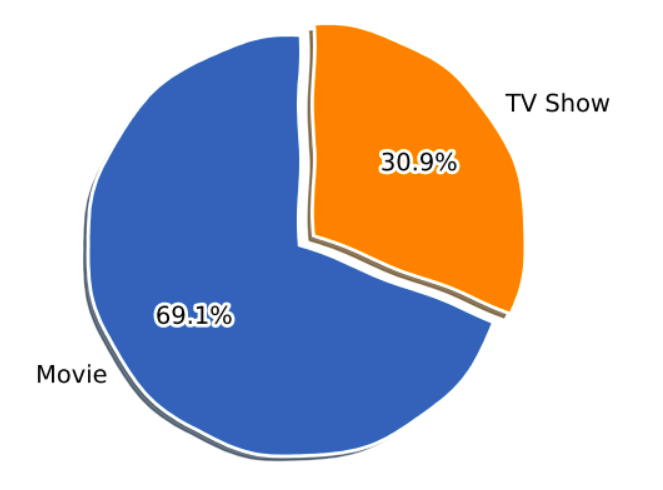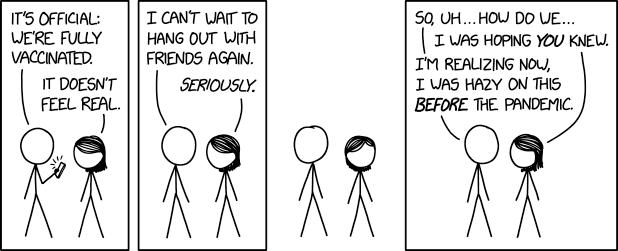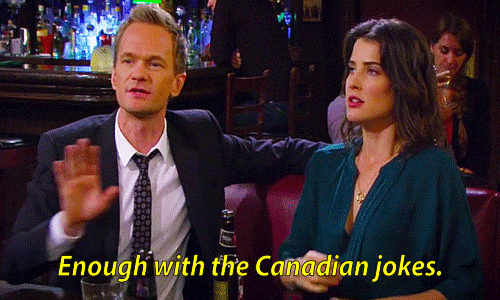# How to make comical visualizations? Explained using Netflix Movie and TV Show dataset. 📺

After you’re done watching a brilliant show or movie on Netflix, does it ever occur to you just how awesome Netflix is for giving you access to this amazing plethora of content? Surely, I’m not alone in this, am I?

One thought leads to another, and before you know it, you’ve made up your mind to do an exploratory data analysis to find out more about who the most popular actors are and which country prefers which genre.

Now, I’ve spent my fair share of time making regular bar plots and pie plots using Python, and while they do a perfect job in conveying the results, I wanted to add a little fun element to this project.

I recently learned that you can create xkcd-like plots in matplotlib, Python’s most popular data viz library, and decided that I should comify all my plots in this project just to make things a little more interesting.

Let’s take a look at what the data has to say!

### The data

I used this dataset, which’s available on Kaggle. It contains 7,787 movie and TV show titles available on Netflix as of 2020.

To start off, I installed the required libraries and read the CSV file.

``````import pandas as pd
import matplotlib.pyplot as plt
plt.rcParams['figure.dpi'] = 200

df = pd.read_csv("../input/netflix-shows/netflix_titles.csv")
df.head()
``````

I also added new features to the dataset that will be used later on in the project.

``````df["date_added"] = pd.to_datetime(df['date_added'])
df['year_added'] = df['date_added'].dt.year.astype('Int64')
df['month_added'] = df['date_added'].dt.month

df['season_count'] = df.apply(lambda x : x['duration'].split(" ") if "Season" in x['duration'] else "", axis = 1)
df['duration'] = df.apply(lambda x : x['duration'].split(" ") if "Season" not in x['duration'] else "", axis = 1)
df.head()
``````

Now we can get to the interesting stuff!

Let me also add that, to XKCDify plots in matplotlib, you just need to engulf all your plotting code within the following block and you’ll be all set:

``````with plt.xkcd():
#all your regular visualization code goes in here
``````### 1. Netflix through the years

First, I thought it would be worth looking at a timeline that depicts the evolution of Netflix over the years.

``````from datetime import datetime

# these go on the numbers below
tl_dates = [
"1997\nFounded",
"1998\nMail Service",
"2003\nGoes Public",
"2007\nStreaming service",
"2016\nGoes Global",
"2021\nNetflix & Chill"
]

tl_x = [1, 2, 4, 5.3, 8,9]

# the numbers go on these
tl_sub_x = [1.5,3,5,6.5,7]

tl_sub_times = [
"1998","2000","2006","2010","2012"
]

tl_text = [
"Netflix.com launched",
"Starts\nPersonal\nRecommendations","Billionth DVD Delivery","Canadian\nLaunch","UK Launch"]

with plt.xkcd():
# Set figure & Axes
fig, ax = plt.subplots(figsize=(15, 4), constrained_layout=True)
ax.set_ylim(-2, 1.75)
ax.set_xlim(0, 10)

# Timeline : line
ax.axhline(0, xmin=0.1, xmax=0.9, c='deeppink', zorder=1)

# Timeline : Date Points
ax.scatter(tl_x, np.zeros(len(tl_x)), s=120, c='palevioletred', zorder=2)
ax.scatter(tl_x, np.zeros(len(tl_x)), s=30, c='darkmagenta', zorder=3)
# Timeline : Time Points
ax.scatter(tl_sub_x, np.zeros(len(tl_sub_x)), s=50, c='darkmagenta',zorder=4)

# Date Text
for x, date in zip(tl_x, tl_dates):
ax.text(x, -0.55, date, ha='center',
fontfamily='serif', fontweight='bold',
color='royalblue',fontsize=12)

# Stemplot : vertical line
levels = np.zeros(len(tl_sub_x))
levels[::2] = 0.3
levels[1::2] = -0.3
markerline, stemline, baseline = ax.stem(tl_sub_x, levels, use_line_collection=True)
plt.setp(baseline, zorder=0)
plt.setp(markerline, marker=',', color='darkmagenta')
plt.setp(stemline, color='darkmagenta')

# Text
for idx, x, time, txt in zip(range(1, len(tl_sub_x)+1), tl_sub_x, tl_sub_times, tl_text):
ax.text(x, 1.3*(idx%2)-0.5, time, ha='center',
fontfamily='serif', fontweight='bold',
color='royalblue', fontsize=11)

ax.text(x, 1.3*(idx%2)-0.6, txt, va='top', ha='center',
fontfamily='serif',color='royalblue')

# Spine
for spine in ["left", "top", "right", "bottom"]:
ax.spines[spine].set_visible(False)

# Ticks
ax.set_xticks([])
ax.set_yticks([])

# Title
ax.set_title("Netflix through the years", fontweight="bold", fontfamily='serif', fontsize=16, color='royalblue')
ax.text(2.4,1.57,"From DVD rentals to a global audience of over 150m people - is it time for Netflix to Chill?", fontfamily='serif', fontsize=12, color='mediumblue')

plt.show()
``````

This plot paints a pretty decent picture of Netflix’s journey. Also, the plot looks hand-drawn because of the `plt.xkcd()` function. Wicked stuff.

### 2. Movies vs TV Shows

Next, I decided to take a look at the ratio of Movies vs TV Shows.

``````col = "type"
grouped = df[col].value_counts().reset_index()
grouped = grouped.rename(columns = {col : "count", "index" : col})

with plt.xkcd():
explode = (0, 0.1)  # only "explode" the 2nd slice (i.e. 'TV Show')

fig1, ax1 = plt.subplots(figsize=(5, 5), dpi=100)
ax1.pie(grouped["count"], explode=explode, labels=grouped["type"], autopct='%1.1f%%',
shadow=True, startangle=90)
ax1.axis('equal')  # Equal aspect ratio ensures that pie is drawn as a circle.

plt.show()
``````The number of TV shows on the platform is less than a third of the total content. So probably, both you and I have better chances of finding a relatively good movie than a TV Show on Netflix. *sighs*.

### 3. Countries with the most content

For my third visualization, I wanted to make a horizontal bar graph that represented the top 25 countries with the most content. The `country` column in the data frame had a few rows that contained more than 1 country (separated by commas).

To handle this, I split the data in the `country` column with `", “` as the separator and then put all the countries into a list called `categories` :

``````from collections import Counter
col = "country"

categories = ", ".join(df[col].fillna("")).split(", ")
counter_list = Counter(categories).most_common(25)
counter_list = [_ for _ in counter_list if _ != ""]
labels = [_ for _ in counter_list]
values = [_ for _ in counter_list]

with plt.xkcd():
fig, ax = plt.subplots(figsize=(10, 10), dpi=100)
y_pos = np.arange(len(labels))
ax.barh(y_pos, values, align='center')
ax.set_yticks(y_pos)
ax.set_yticklabels(labels)
ax.invert_yaxis()  # labels read top-to-bottom
ax.set_xlabel('Content')
ax.set_title('Countries with most content')

plt.show()
``````

Some overall thoughts after looking at the plot above:

• The vast majority of content on Netflix is from the United States (quite obvious).
• Even though Netflix launched quite late in India (in 2016), it’s already in the second position right after the US. So, India is a big market for Netflix.
• I’m going to look for content from Thailand on Netflix, now that I know that it’s there on the platform, brb.

### 4. Popular directors and actors

To take a look at the popular directors and actors, I decided to plot a figure (each) with six subplots from the top six countries with the most content and make horizontal bar charts for each subplot. Take a look at the plots below and read that first line again.#### a. Popular directors:

``````from collections import Counter
from matplotlib.pyplot import figure
import math
​
colours = ["orangered", "mediumseagreen", "darkturquoise", "mediumpurple", "deeppink", "indianred"]
countries_list = ["United States", "India", "United Kingdom", "Japan", "France", "Canada"]
col = "director"
​
with plt.xkcd():
figure(num=None, figsize=(20, 8))
x=1
for country in countries_list:
country_df = df[df["country"]==country]
categories = ", ".join(country_df[col].fillna("")).split(", ")
counter_list = Counter(categories).most_common(6)
counter_list = [_ for _ in counter_list if _ != ""]
labels = [_ for _ in counter_list][::-1]
values = [_ for _ in counter_list][::-1]
if max(values)<10:
values_int = range(0, math.ceil(max(values))+1)
else:
values_int = range(0, math.ceil(max(values))+1, 2)
plt.subplot(2, 3, x)
plt.barh(labels,values, color = colours[x-1])
plt.xticks(values_int)
plt.title(country)
x+=1
plt.suptitle('Popular Directors with the most content')
plt.tight_layout()
plt.show()
``````

#### b. Popular actors:

``````col = "cast"

with plt.xkcd():
figure(num=None, figsize=(20, 8))
x=1
for country in countries_list:
df["from_country"] = df['country'].fillna("").apply(lambda x : 1 if country.lower() in x.lower() else 0)
small = df[df["from_country"] == 1]
cast = ", ".join(small['cast'].fillna("")).split(", ")
tags = Counter(cast).most_common(11)
tags = [_ for _ in tags if "" != _]
labels, values = [_+"  " for _ in tags][::-1], [_ for _ in tags][::-1]
if max(values)<10:
values_int = range(0, math.ceil(max(values))+1)
elif max(values)>=10 and max(values)<=20:
values_int = range(0, math.ceil(max(values))+1, 2)
else:
values_int = range(0, math.ceil(max(values))+1, 5)
plt.subplot(2, 3, x)
plt.barh(labels,values, color = colours[x-1])
plt.xticks(values_int)
plt.title(country)
x+=1
plt.suptitle('Popular Actors with the most content')
plt.tight_layout()
plt.show()
``````

### 5. Some of the oldest movies and TV shows

I thought it would be quite interesting to look at the oldest movies and TV shows that are available on Netflix and how long back they’re dated.

#### a. Oldest movies:

``````small = df.sort_values("release_year", ascending = True)
small = small[small['duration'] != ""].reset_index()
small[['title', "release_year"]][:15]
``````

#### b. Oldest TV shows:

``````small = df.sort_values("release_year", ascending = True)
small = small[small['season_count'] != ""].reset_index()
small = small[['title', "release_year"]][:15]
small
``````

Woah, Netflix has some realllyyy old movies and TV shows, some even released more than 80 years ago. Have you watched any of these?

(Fun fact: When he began implementing Python, Guido van Rossum was also reading the published scripts from “Monty Python’s Flying Circus”, a BBC comedy series from the 1970s (that was added on Netflix in 2018). Van Rossum thought he needed a name that was short, unique, and slightly mysterious, so he decided to call the language Python.)

### 6. Does Netflix have the latest content?

Yes, Netflix is cool and all for having content from a century ago, but does it also have the latest movies and TV shows? To find this out, first I calculated the difference between the date on which the content was added on Netflix and the release year of that content.

``````df["year_diff"] = df["year_added"]-df["release_year"]
``````

Then, I created a scatter plot with x-axis as the year difference and y-axis as the number of movies/TV shows:

``````col = "year_diff"
only_movies = df[df["duration"]!=""]
only_shows = df[df["season_count"]!=""]
grouped1 = only_movies[col].value_counts().reset_index()
grouped1 = grouped1.rename(columns = {col : "count", "index" : col})
grouped1 = grouped1.dropna()
grouped1 = grouped1.head(20)
grouped2 = only_shows[col].value_counts().reset_index()
grouped2 = grouped2.rename(columns = {col : "count", "index" : col})
grouped2 = grouped2.dropna()
grouped2 = grouped2.head(20)

with plt.xkcd():
figure(num=None, figsize=(8, 5))
plt.scatter(grouped1[col], grouped1["count"], color = "hotpink")
plt.scatter(grouped2[col], grouped2["count"], color = '#88c999')
values_int = range(0, math.ceil(max(grouped1[col]))+1, 2)
plt.xticks(values_int)
plt.xlabel("Difference between the year when the content has been\n added on Netflix and the realease year")
plt.ylabel("Number of Movies/TV Shows")
plt.legend(["Movies", "TV Shows"])
plt.tight_layout()
plt.show()
``````

As you can see, the majority of the content on Netflix has been added within a year of its release date. So, Netflix does have the latest content most of the time!

If you’re still here, here’s an xkcd comic for you, you’re welcome.### 7. What kind of content is Netflix focusing upon?

I also wanted to explore the `rating` column and compare the amount of content that Netflix has been producing for kids, teens, and adults and if their focus has shifted from one group to the other over the years.

To achieve this, first I took a look at the unique ratings in the data frame:

`print(df['rating'].unique())`

Output:

`['TV-MA' 'R' 'PG-13' 'TV-14' 'TV-PG' 'NR' 'TV-G' 'TV-Y' nan 'TV-Y7' 'PG' 'G' 'NC-17' 'TV-Y7-FV' 'UR']`

Then, I classified the ratings according to the groups (namely — Little Kids, Older Kids, Teens and Mature) they fall into and changed their values in the `rating` column to their group names.

``````ratings_list = ['TV-MA', 'R', 'PG-13', 'TV-14', 'TV-PG', 'TV-G', 'TV-Y', 'TV-Y7', 'PG', 'G', 'NC-17', 'TV-Y7-FV']
ratings_group_list = ['Little Kids', 'Older Kids', 'Teens', 'Mature']
ratings_dict={
'TV-G': 'Little Kids',
'TV-Y': 'Little Kids',
'G': 'Little Kids',
'TV-PG': 'Older Kids',
'TV-Y7': 'Older Kids',
'PG': 'Older Kids',
'TV-Y7-FV': 'Older Kids',
'PG-13': 'Teens',
'TV-14': 'Teens',
'TV-MA': 'Mature',
'R': 'Mature',
'NC-17': 'Mature'
}
for rating_val, rating_group in ratings_dict.items():
df.loc[df.rating == rating_val, "rating"] = rating_group
``````

Finally, I made line plots with year on the x-axis and content count on the y-axis.

``````df['rating_val']=1
x=0
labels=['kinda\nless', 'not so\nbad', 'holyshit\nthat\'s too\nmany']

with plt.xkcd():
for r in ratings_group_list:
grouped = df[df['rating']==r]
year_df = grouped.groupby(['year_added']).sum()
year_df.reset_index(level=0, inplace=True)
plt.plot(year_df['year_added'], year_df['rating_val'], color=colours[x], marker='o')
values_int = range(2008, math.ceil(max(year_df['year_added']))+1, 2)
plt.yticks([200, 600, 1000], labels)
plt.xticks(values_int)
plt.title('Count of shows and movies that Netflix\n has been producing for different audiences', fontsize=12)
plt.xlabel('Year', fontsize=14)
plt.ylabel('Content Count', fontsize=14)
x+=1
plt.legend(ratings_group_list)
plt.tight_layout()
plt.show()
``````

Okay, so the content count for mature audiences on Netflix is way more than the other groups. Another interesting observation is that there was a surge in the count of content produced for Little Kids from 2019–2020 whereas the content for Older Kids, Teens and Mature Audiences decreased during that time period.

### 8. Top Genres (Countrywise)

``````col = "listed_in"
colours = ["violet", "cornflowerblue", "darkseagreen", "mediumvioletred", "blue", "mediumseagreen", "darkmagenta", "darkslateblue", "seagreen"]
countries_list = ["United States", "India", "United Kingdom", "Japan", "France", "Canada", "Spain", "South Korea", "Germany"]

with plt.xkcd():
figure(num=None, figsize=(20, 8))
x=1
for country in countries_list:
df["from_country"] = df['country'].fillna("").apply(lambda x : 1 if country.lower() in x.lower() else 0)
small = df[df["from_country"] == 1]
genre = ", ".join(small['listed_in'].fillna("")).split(", ")
tags = Counter(genre).most_common(3)
tags = [_ for _ in tags if "" != _]
labels, values = [_+"  " for _ in tags][::-1], [_ for _ in tags][::-1]
if max(values)>200:
values_int = range(0, math.ceil(max(values)), 100)
elif max(values)>100 and max(values)<=200:
values_int = range(0, math.ceil(max(values))+50, 50)
else:
values_int = range(0, math.ceil(max(values))+25, 25)
plt.subplot(3, 3, x)
plt.barh(labels,values, color = colours[x-1])
plt.xticks(values_int)
plt.title(country)
x+=1
plt.suptitle('Top Genres')
plt.tight_layout()
plt.show()
``````

Key takeaways from this plot:

• Dramas and Comedies are the most popular genres in almost every country.

• Japan watches a LOT of anime!

• Romantic TV Shows and TV Dramas are big in South Korea. (I’m addicted to K-Dramas too, btw)

• Children and Family Movies are the third most popular genre in Canada.### 9. Word Clouds

I finally ended the project with two word clouds — first, a word cloud for the `description` column and a second one for the `title` column.

#### a. Word Cloud for Description:

``````from wordcloud import WordCloud
import random
from PIL import Image
import matplotlib

# Custom colour map based on Netflix palette
cmap = matplotlib.colors.LinearSegmentedColormap.from_list("", ['#221f1f', '#b20710'])

text = str(list(df['description'])).replace(',', '').replace('[', '').replace("'", '').replace(']', '').replace('.', '')

mask = np.array(Image.open('../input/finallogo/New Note.png'))

wordcloud = WordCloud(background_color = 'white', width = 500, height = 200,colormap=cmap, max_words = 150, mask = mask).generate(text)

plt.figure( figsize=(5,5))
plt.imshow(wordcloud, interpolation = 'bilinear')
plt.axis('off')
plt.tight_layout(pad=0)
plt.show()
``````

Live, love, life, friend, family, world and find are some of the most frequent words to appear in the descriptions of movies and shows. Another interesting thing is that the words — one, two, three and four — all appear in the word cloud.

#### b. Word Cloud for Title:

``````cmap = matplotlib.colors.LinearSegmentedColormap.from_list("", ['#221f1f', '#b20710'])

text = str(list(df['title'])).replace(',', '').replace('[', '').replace("'", '').replace(']', '').replace('.', '')

mask = np.array(Image.open('../input/finallogo/New Note.png'))

wordcloud = WordCloud(background_color = 'white', width = 500, height = 200,colormap=cmap, max_words = 150, mask = mask).generate(text)

plt.figure( figsize=(5,5))
plt.imshow(wordcloud, interpolation = 'bilinear')
plt.axis('off')
plt.tight_layout(pad=0)
plt.show()
``````

Do you see Christmas right at the center of this word cloud? Seems like there is an abundance of Christmas movies on Netflix. Other popular words are — Love, World, Man, Life, Story, Live, Secret, Girl, Boy, American, Game, Night, Last, Time and Day.

### And that’s it!

It just took me a few hours to complete this project and I am now able to see everything that Netflix does in a new way. I think this is the funnest project I’ve done so far and I’d definitely like to expand on this by building a recommendation system next. Working on projects like these reminds me that data science really is so cool.

If you have any questions/feedback or would just like to chat, you can reach out to me on Twitter or LinkedIn.

18 Likes

Wow! What a great read, Paridhi!This is such a cool project idea with even cooler visualisations. I love the humour you’ve sprinkled throughout your article!

Amazing work!!1 Like

Nice project and article @theparidhi0! I know you’ve been working a lot on the writing side of things and it’s paying off.

2 Likes

Thank you so much Nityesh!1 Like

Thank you so much Michael. This means a lot coming from you. Your advice has been invaluable and that’s helped me have fun with the writing process so much.

1 Like

Nice…
I also did EDA on a Netflix dataset (which had millions of ratings by random thousands of Netflix customers) recently, and your EDA kinda completes the picture for me.1 Like

Hi Archit, thank you so much!Fun work Paridhi, Kudos!!

1 Like

The best part of this entire project, is it’s simplicity. Just pick up a dataset, analyze and plot the analysis, et voila` an awesome project!

2 Likes

Exactly! It’s so simple yet so fun!1 Like

Such an amazing piece of work.
When visualization is done with great humour, detailed communication and bits of storytelling, driving the point home will be easier.

Kudos Paridhi on this amazing work you’ve done.2 Likes

Thank you for your kind words @info.victoromondiThis is amazing! Thanks you!!

1 Like

Thank you for saying this @bvalgard !